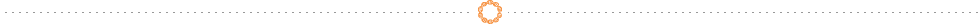Skip to content

# Complimentary Body Health Scan

Bio impedance analysis (BIA) is a commonly used method for estimating body composition, and in particular body fat. It determines the electrical impedance, or opposition to the flow of an electric current through body tissues which can then be used to calculate an estimate of total body water (TBW). TBW can be used to estimate fat-free body mass and, by difference with body weight, body fat.

The impedance of cellular tissue can be modeled as a resistor (representing the extracellular path) in parallel with a resistor and capacitor in series (representing the intracellular path). This results in a change in impedance versus the frequency used in the measurement. The impedance measurement is generally measured from the wrist to the contralateral ankle and uses either two or four electrodes. A small current on the order of 1-10 µA is passed between two electrodes, and the voltage is measured between the same (for a two electrode configuration) or between the other two electrodes.